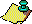Contents Next: The Septic Iteration Up: Approximations to via the Previous: The Quadratic and

# The Cubic Iteration

We summarise the results of [6,7] and how they correspond to the case p=3. Define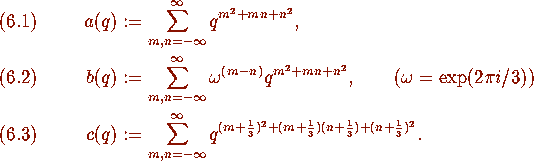Then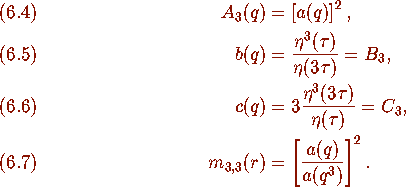We also mention the identityThis equation was known to Ramanujan [3, p. 199,]. See  for a proof using theta-functions.

We have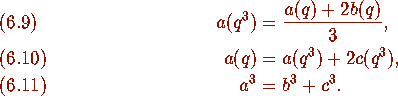As usual we let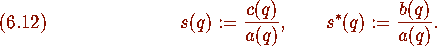Then, from (6.9)--(6.12) we have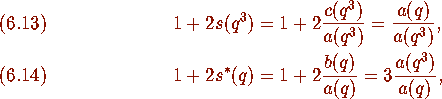and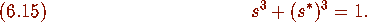Now, from (6.7), (6.13), (6.14) we have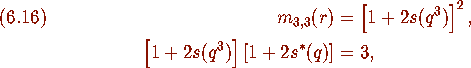and, by (3.18), (6.16) we have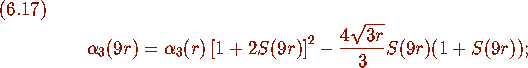where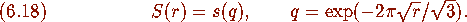From (3.19), (3.20) we know that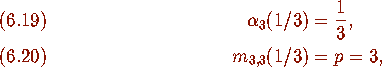and so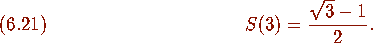By letting,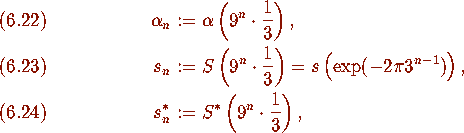we have the following

### Theorem 6.1

(Borwein and Borwein [6, Iteration 3.2, p. 699,]) Define sequences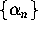,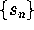, and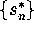by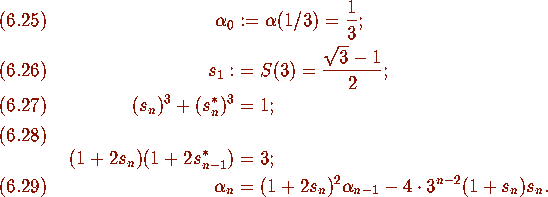Then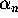converges cubically to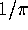.Contents Next: The Septic Iteration Up: Approximations to via the Previous: The Quadratic and